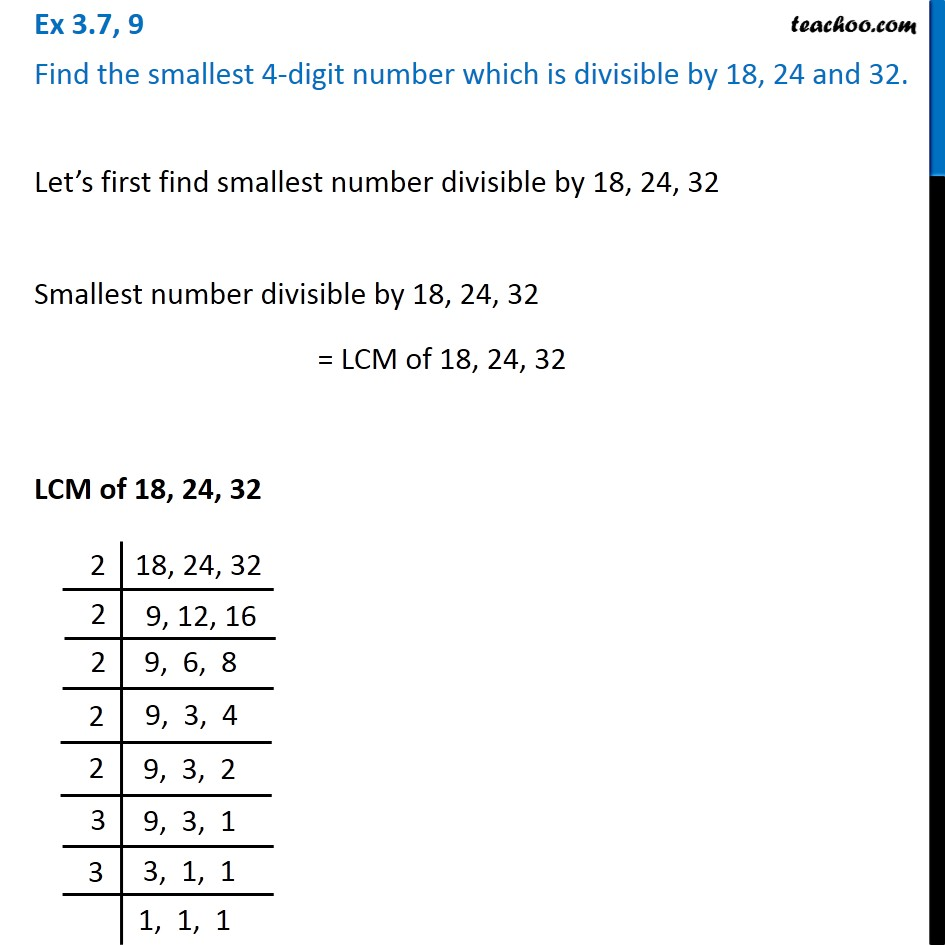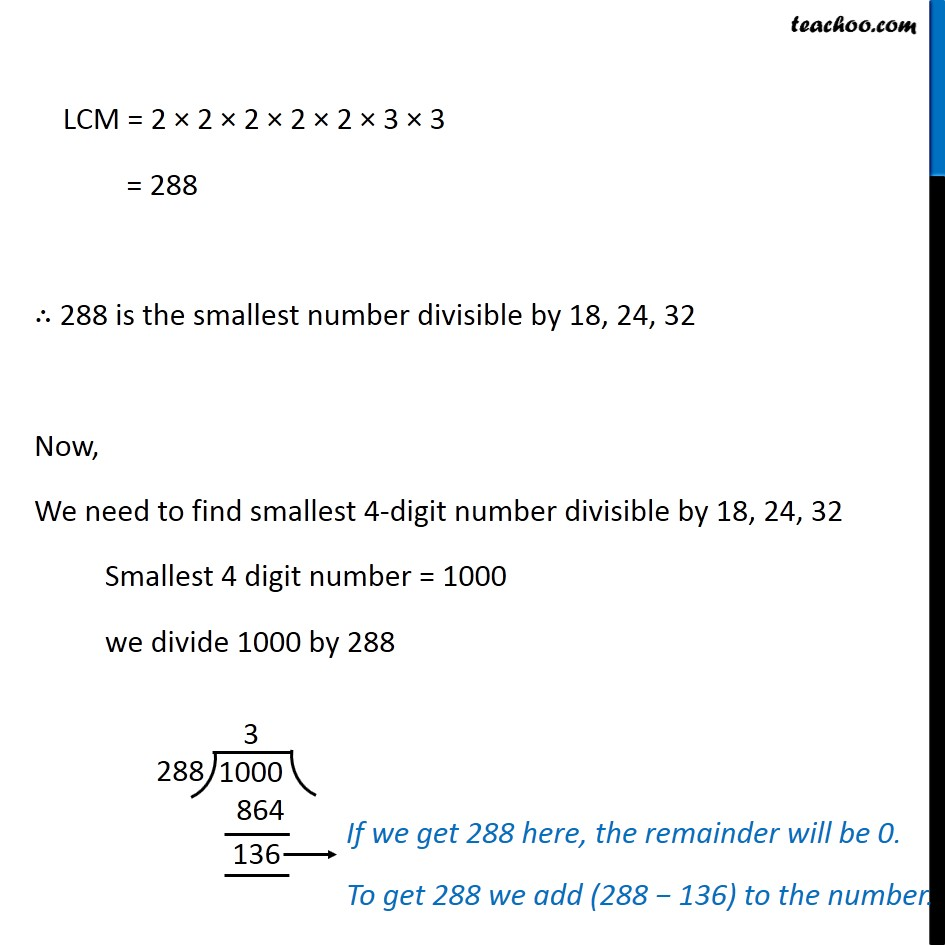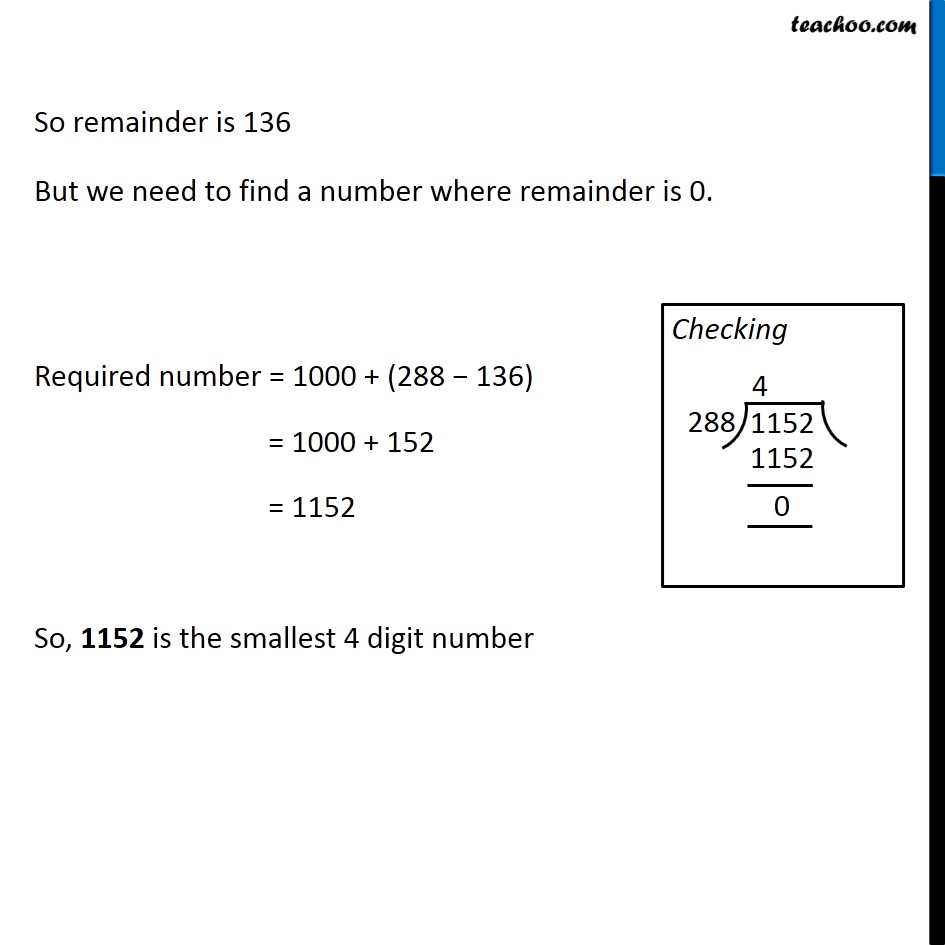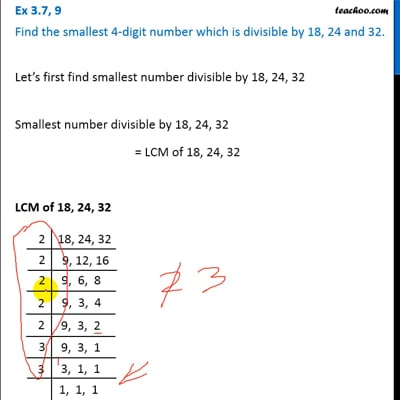Statement questions on LCM

Chapter 3 Class 6 Playing with Numbers
Concept wiseThis video is only available for Teachoo black users

### Transcript

Let’s first find smallest number divisible by 18, 24, 32 Smallest number divisible by 18, 24, 32 = LCM of 18, 24, 32 LCM of 18, 24, 32 LCM = 2 × 2 × 2 × 2 × 2 × 3 × 3 = 288 ∴ 288 is the smallest number divisible by 18, 24, 32 Now, We need to find smallest 4-digit number divisible by 18, 24, 32 Smallest 4 digit number = 1000 we divide 1000 by 288 So remainder is 136 But we need to find a number where remainder is 0. Required number = 1000 + (288 − 136) = 1000 + 152 = 1152 So, 1152 is the smallest 4 digit number